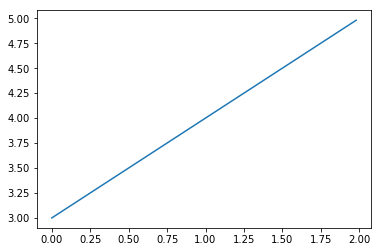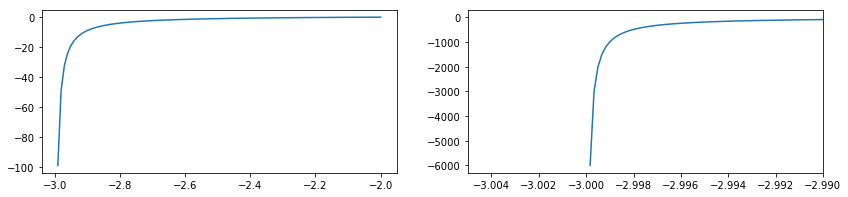## Limit of a Function

A function limit, roughly speaking, describes the behavior of a function around a specific value. Limits play a role in the definition of the derivative and function continuity and are also used in the convergent sequences.

Before getting to the precise definition of a limit, we can investigate limit of a function by plotting it and examining the area around the limit value.

For example, consider the limit (taken from James Stewart's Calculus Early Transcendentals, Exercise 11, page 107)

$$\lim_{x\to 2} \frac{x^2 - x - 6}{x - 2}$$

We can plot this limit using the matplotlib.pylot module. The numpy library is also imported for some convenience functions.

In :
import numpy as np
import matplotlib.pyplot as plt

In :
def f(x):
return (x ** 2 + x - 6) / (x - 2)


Get a set of values for $x$ using the linspace function.

In :
xvals = np.linspace(0, 2, 100, False)


Then plot the function using matplotlib.

In :
plt.plot(xvals, f(xvals))
plt.show()The plot shows that as $x$ gets closer to $2$, the function gets close to $5$. One way to verify this is to look closer at the values of the function around the limit.

In :
xvals2 = np.linspace(1.90, 2, 100, False)

In :
f(xvals2)

Out:
array([ 4.9  ,  4.901,  4.902,  4.903,  4.904,  4.905,  4.906,  4.907,
4.908,  4.909,  4.91 ,  4.911,  4.912,  4.913,  4.914,  4.915,
4.916,  4.917,  4.918,  4.919,  4.92 ,  4.921,  4.922,  4.923,
4.924,  4.925,  4.926,  4.927,  4.928,  4.929,  4.93 ,  4.931,
4.932,  4.933,  4.934,  4.935,  4.936,  4.937,  4.938,  4.939,
4.94 ,  4.941,  4.942,  4.943,  4.944,  4.945,  4.946,  4.947,
4.948,  4.949,  4.95 ,  4.951,  4.952,  4.953,  4.954,  4.955,
4.956,  4.957,  4.958,  4.959,  4.96 ,  4.961,  4.962,  4.963,
4.964,  4.965,  4.966,  4.967,  4.968,  4.969,  4.97 ,  4.971,
4.972,  4.973,  4.974,  4.975,  4.976,  4.977,  4.978,  4.979,
4.98 ,  4.981,  4.982,  4.983,  4.984,  4.985,  4.986,  4.987,
4.988,  4.989,  4.99 ,  4.991,  4.992,  4.993,  4.994,  4.995,
4.996,  4.997,  4.998,  4.999])

We can also use SymPy's limit function to calculate the limit.

In :
from sympy import symbols, limit, sin, cos, init_printing

In [ ]:
x = symbols('x')
init_printing()

In [ ]:
limit((x ** 2 + x - 6) / (x - 2), x, 2)


Using this information, we can construct a more precise definition of a limit.

## Definition of a Limit¶

Limits are typically denoted as:

$$\lim_{x\to a} \space f(x) = L$$

Or, alternatively:

$$f(x) \rightarrow L, \qquad x \rightarrow a$$

In plain language, we can state the limit as, "the limit of the a function $f(x)$ as $x$ approaches $a$ is equal to $L$. For example, if we were considering the limit:

$$\lim_{x \to 2} \space f(x) = 5$$

We can state it as, "the limit of the function $f(x)$ as $x$ approaches $2$ is equal to $5$.

## One-Sided Limits¶

One-sided limits are used to express a limit as it approaches $a$ from a particular direction. The notation is similar to the limit seen above but with a slight change to indicate which direction $x$ is headed.

$$\lim_{x \to a^+} \space f(x) = L, \qquad \lim_{x \to a^-} \space f(x) = L$$

The notation $x \to a^+$ states we are only interested in values of $x$ that are greater than $a$. Similarly, the notation $x \to a^-$ denotes our desire to investigate values of $x$ less than $a$. These one-sided limits are also referred to as the "right-hand limit" and "left-hand limit", respectively.

For example, consider the function:

$$\lim_{x \to -3^+} \space \frac{x + 2}{x + 3}$$

The $-3^+$ notation tells us we are only interested values greater than -3; thus the limit is a right-hand limit.

The function is not defined for $x = 3$; therefore we are dealing with an infinite limit. We can see the behavior of the function as $x$ approaches $-3$ by plotting.

In :
def f2(x):
return (x + 2) / (x + 3)

In :
xvals2 = np.linspace(-2, -3, 100, False)
xvals3 = np.linspace(-2.5, -3, 3000, False)

In :
plt.figure(1, figsize=(14,3))
plt.subplot(121)
plt.plot(xvals2, f2(xvals2))

plt.subplot(122)
plt.plot(xvals3, f2(xvals3))
plt.xlim((-3.005, -2.99))
plt.show()Both graphs approach $x = -3$ from the right and we can see the function quickly drop off as it gets closer to its limit. The graph on the right is a magnified representation of the graph on the left to better illustrate the infinite limit. Therefore, the limit of the function is $-\infty$, which we can confirm with SymPy.

In :
limit((x + 2) / (x + 3), x, -3)

Out:
$$-\infty$$